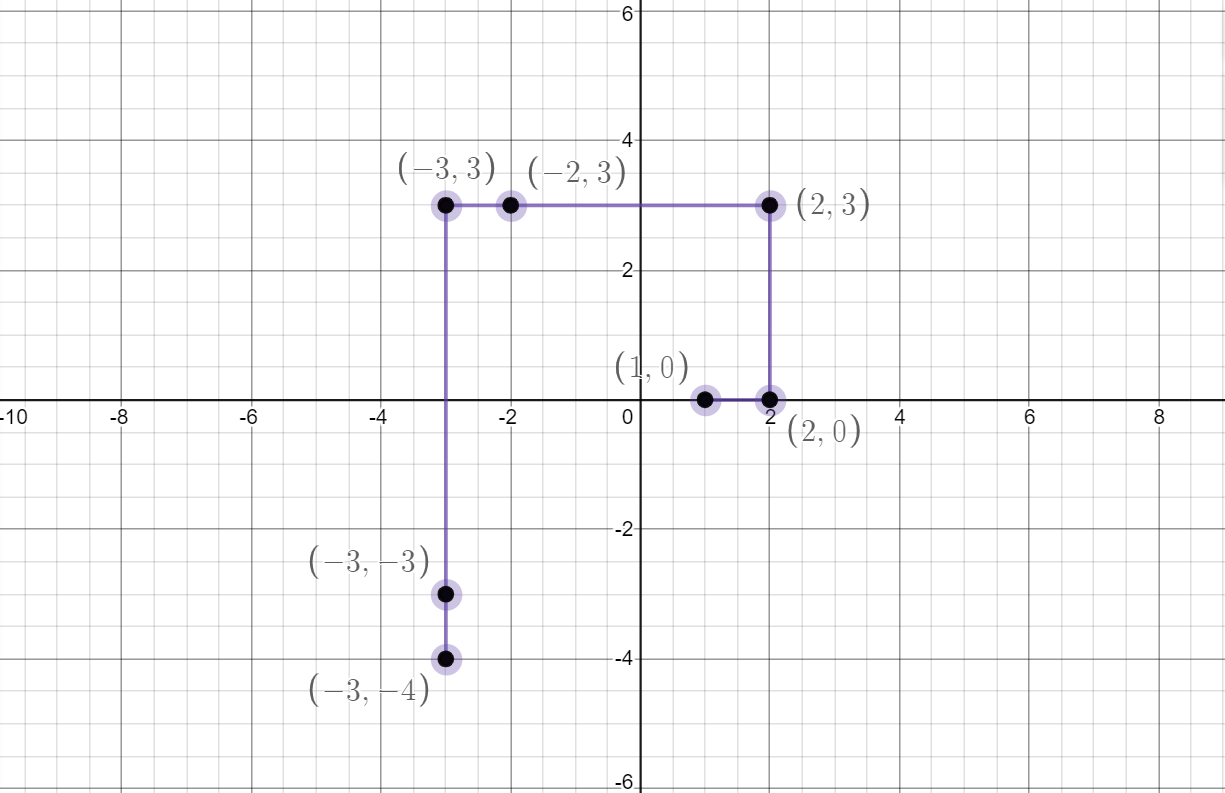# CCL3 - Editorial

Author: bhavya_dhingra
Tester: Ankur1903
Editorialists: hasan8130, deshraja1

EASY

## Prerequisites:

Binary Search, Sieve Algorithm

## Problem:

Given that the ray takes the first turn after covering 2 miles (a_1 = 2), for a given unit of distance covered, find out the x and y coordinates.

## Explanation:

Morty’s Ray will travel in a Rectilinear motion until it either finds Rick or covers a distance a_i such that a_i is the smallest number greater than a_i-1 and coprime to all the numbers from a_1 to a_i−1.This simply implies that a_i is the ith prime number. So if we draw the figure for question we can clearly see a spiral forming with prime numbers as its edge points.In the diagram we can see that, a_1=2 so we start from 2 and the coordinates at 2 are
(0,0). Now since 2 is a prime number, it will move 2 steps and at coordinates (2,0) it will take a left turn and reach 3 whose coordinates are (2,3), then it goes to (-2,3) at 4, since 4 is not a prime number, therefore it goes straight to 5 at coordinates
(-3,3), now 5 is a prime number hence it takes left turn and moves to 6 having coordinates (-3,-3), then since 6 is not a prime number so it moves to 7 which has coordinates (-3,-4) and so on.

A simple and a brute force solution will be to calculate the coordinates of N at each query by simply iterating x and y by 1 and i + 1(in case of turns) but this will require O( Q * N ) Time.

### Prime Preprocessing

As a brute force solution will not be fast enough we can reduce it’s time by precomputing all the prime numbers from 1 to 10^6 using Sieve of Eratosthenes(This will require O(n) Time ) and store them in a Array or Vector named Prime, In addition to that we will create a Coordinates array to store coordinate of i’th prime number at i’th index.

### Computing Coordinates

Coordinates array of pair of int, int can be computed by observing the direction of the ray at that instance since there are 4 possible directions,

we can define directions as 0,1,2,3 as i % 4 as :

if i % 4 == 0 then x += prime[i]

if i % 4 == 1 then y += prime[i]

if i % 4 == 2 then x -= prime[i]

if i % 4 == 3 then y -= prime[i]

then these x,y will be stored in the coordinates array as a location of i’th prime. This can be seen through simple observation.

### Processing The Queries

After the preprocessing is done we can find every query in O(logN) time by searching the position of the nearest prime using binary search. Index of Nearest Prime will be stored as X. Now, if X == N then return coordinates of prime N. If Not then, x,y will be coordinates of X and these x,y will be modified as

if X % 4 == 0 then y += N

if X % 4 == 1 then x -= N

if X % 4 == 2 then y -= N

if X % 4 == 3 then x += N

And finally these x,y will be the required coordinates.

## Solution:

Setter's Solution
`````` #include <iostream>
#include <string>
#include <set>
#include <map>
#include <stack>
#include <queue>
#include <vector>
#include <utility>
#include <iomanip>
#include <sstream>
#include <bitset>
#include <cstdlib>
#include <iterator>
#include <algorithm>
#include <cstdio>
#include <cctype>
#include <cmath>
#include <math.h>
#include <ctime>
#include <cstring>
#include <unordered_set>
#include <unordered_map>
using namespace std;
#define sync {ios_base ::sync_with_stdio(false); cin.tie(NULL); cout.tie(NULL);}
#define rep(n) for(int i = 0;i<n;i++)
#define rep1(a,b) for(int i = a;i<b;i++)
#define int long long int
#define mod 1000000007

bool prime;
vector<int> primes;
vector<pair<int,int>> coor;
void primesieve()
{
int n = 1e6;

memset(prime, true, sizeof(prime));

for (int p=2; p*p<=n; p++)
{
if (prime[p] == true)
{
for (int i=p*p; i<=n; i += p)
prime[i] = false;
}
}

rep1(2,1e6)
{
if(prime[i])
{
primes.push_back(i);
}
}

int x = 0;
int y = 0;
rep(primes.size())
{
if(i%4==0)
{
x+=primes[i];
}
else if(i%4==1)
{
y+=primes[i];
}
else if(i%4==2)
{
x-=primes[i];
}
else
{
y-=primes[i];
}

coor.push_back({x,y});
}
}

int n;

void solve()
{
cin>>n;
if(n==1)
{
// cout<<1<<' '<<0;
return;
}

auto t = lower_bound(primes.begin(),primes.end(),n);
int temp = t-primes.begin();
if(primes[temp]!=n)
{
temp--;
}

int x = coor[temp].first;
int y = coor[temp].second;

if(primes[temp]==n)
{
cout<<x<<' '<<y;
return;
}
if(temp%4==0)
{
y+=n;
}
else if(temp%4==1)
{
x-=n;
}
else if(temp%4==2)
{
y-=n;
}
else
{
x+=n;
}

cout<<x<<' '<<y;

}

int32_t main()
{
// #ifndef ONLINE_JUDGE
// 	freopen("Inp1.txt","r",stdin);
// 	freopen("Op1.txt","w",stdout);
// #endif
// clock_t time_req;
// time_req = clock();
sync;
primesieve();
int t = 1;
cin>>t;
while(t--){
solve();
cout<<"\n";
}
// cout << "Time taken: "<< (float)time_req/CLOCKS_PER_SEC << " seconds" << endl;
return 0;
}
``````
3 Likes